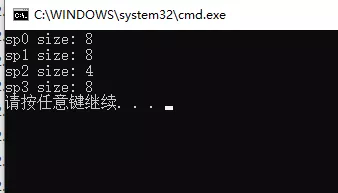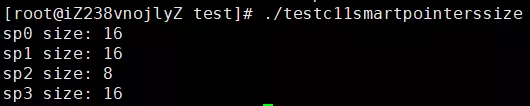# 详解 C++ 11 中的智能指针

• 2021-01-11
• 浏览 (311)

## 详解 C++ 11 中的智能指针

C/C++ 语言最为人所诟病的特性之一就是存在内存泄露问题，因此后来的大多数语言都提供了内置内存分配与释放功能，有的甚至干脆对语言的使用者屏蔽了内存指针这一概念。这里不置贬褒，手动分配内存与手动释放内存有利也有弊，自动分配内存和自动释放内存亦如此，这是两种不同的设计哲学。有人认为，内存如此重要的东西怎么能放心交给用户去管理呢？而另外一些人则认为，内存如此重要的东西怎么能放心交给系统去管理呢？在 C/C++ 语言中，内存泄露的问题一直困扰着广大的开发者，因此各类库和工具的一直在努力尝试各种方法去检测和避免内存泄露，如 boost，智能指针技术应运而生。

#### C++ 98⁄03 的尝试——std::auto_ptr

std::auto_ptr 的基本用法如下代码所示：

``````#include <memory>

int main()
{
//初始化方式1
std::auto_ptr<int> sp1(new int(8));
//初始化方式2
std::auto_ptr<int> sp2;
sp2.reset(new int(8));

return 0;
}
``````

sp 是 smart pointer（智能指针）的简写。

std::auto_ptr 真正让人容易误用的地方是其不常用的复制语义，即当复制一个 std::auto_ptr 对象时（拷贝复制或 operator = 复制），原对象所持有的堆内存对象也会转移给复制出来的对象。示例代码如下：

``````#include <iostream>
#include <memory>

int main()
{
//测试拷贝构造
std::auto_ptr<int> sp1(new int(8));
std::auto_ptr<int> sp2(sp1);
if (sp1.get() != NULL)
{
std::cout << "sp1 is not empty." << std::endl;
}
else
{
std::cout << "sp1 is empty." << std::endl;
}

if (sp2.get() != NULL)
{
std::cout << "sp2 is not empty." << std::endl;
}
else
{
std::cout << "sp2 is empty." << std::endl;
}

//测试赋值构造
std::auto_ptr<int> sp3(new int(8));
std::auto_ptr<int> sp4;
sp4 = sp3;
if (sp3.get() != NULL)
{
std::cout << "sp3 is not empty." << std::endl;
}
else
{
std::cout << "sp3 is empty." << std::endl;
}

if (sp4.get() != NULL)
{
std::cout << "sp4 is not empty." << std::endl;
}
else
{
std::cout << "sp4 is empty." << std::endl;
}

return 0;
}
``````

``````[root@iZ238vnojlyZ testx]# g++ -g -o test_auto_ptr test_auto_ptr.cpp
[root@iZ238vnojlyZ testx]# ./test_auto_ptr
sp1 is empty.
sp2 is not empty.
sp3 is empty.
sp4 is not empty.
``````

``````std::vector<std::auto_ptr<int>> myvectors;
``````

boost 还有 scoped_ptr，C++11 并没有全部照搬，而是选择了三个最实用的指针类型。在 C++11 中可以通过 std::unique_ptr 达到与 boost::scoped_ptr 一样的效果。

#### std::unique_ptr

std::unique_ptr 对其持有的堆内存具有唯一拥有权，也就是说引用计数永远是 1，std::unique_ptr 对象销毁时会释放其持有的堆内存。可以使用以下方式初始化一个 std::unique_ptr 对象：

``````//初始化方式1
std::unique_ptr<int> sp1(new int(123));

//初始化方式2
std::unique_ptr<int> sp2;
sp2.reset(new int(123));

//初始化方式3
std::unique_ptr<int> sp3 = std::make_unique<int>(123);
``````

``````template<typename T, typename... Ts>
std::unique_ptr<T> make_unique(Ts&& ...params)
{
return std::unique_ptr<T>(new T(std::forward<Ts>(params)...));
}
``````

``````template <class T>
class unique_ptr
{
//省略其他代码...

//拷贝构造函数和赋值运算符被标记为delete
unique_ptr(const unique_ptr&) = delete;
unique_ptr& operator=(const unique_ptr&) = delete;
};
``````

``````std::unique_ptr<int> sp1(std::make_unique<int>(123));;

//以下代码无法通过编译
//std::unique_ptr<int> sp2(sp1);
std::unique_ptr<int> sp3;
//以下代码无法通过编译
//sp3 = sp1;
``````

``````#include <memory>

std::unique_ptr<int> func(int val)
{
std::unique_ptr<int> up(new int(val));
return up;
}

int main()
{
std::unique_ptr<int> sp1 = func(123);

return 0;
}
``````

``````#include <memory>

int main()
{
std::unique_ptr<int> sp1(std::make_unique<int>(123));

std::unique_ptr<int> sp2(std::move(sp1));

std::unique_ptr<int> sp3;
sp3 = std::move(sp2);

return 0;
}
``````

``````template<typename T, typename Deletor>
class unique_ptr
{
//其他函数省略...
public:
unique_ptr(unique_ptr&& rhs)
{
this->m_pT = rhs.m_pT;
//源对象释放
rhs.m_pT = nullptr;
}

unique_ptr& operator=(unique_ptr&& rhs)
{
this->m_pT = rhs.m_pT;
//源对象释放
rhs.m_pT = nullptr;
return *this;
}

private:
T*    m_pT;
};
``````

std::unique_ptr 不仅可以持有一个堆对象，也可以持有一组堆对象，示例如下：

``````#include <iostream>
#include <memory>

int main()
{
//创建10个int类型的堆对象
//形式1
std::unique_ptr<int[]> sp1(new int);

//形式2
std::unique_ptr<int[]> sp2;
sp2.reset(new int);
//形式3
std::unique_ptr<int[]> sp3(std::make_unique<int[]>(10));

for (int i = 0; i < 10; ++i)
{
sp1[i] = i;
sp2[i] = i;
sp3[i] = i;
}

for (int i = 0; i < 10; ++i)
{
std::cout << sp1[i] << ", " << sp2[i] << ", " << sp3[i] << std::endl;
}

return 0;
}
``````

``````[root@myaliyun testmybook]# g++ -g -o test_unique_ptr_with_array test_unique_ptr_with_array.cpp -std=c++17
[root@myaliyun testmybook]# ./test_unique_ptr_with_array
0, 0, 0
1, 1, 1
2, 2, 2
3, 3, 3
4, 4, 4
5, 5, 5
6, 6, 6
7, 7, 7
8, 8, 8
9, 9, 9
``````

std::shared_ptrstd::weak_ptr 也可以持有一组堆对象，用法与 std::unique_ptr 相同，下文不再赘述。

``````#include <iostream>
#include <memory>

class Socket
{
public:
Socket()
{

}

~Socket()
{

}

//关闭资源句柄
void close()
{

}
};

int main()
{
auto deletor = [](Socket* pSocket) {
//关闭句柄
pSocket->close();
//TODO: 你甚至可以在这里打印一行日志...
delete pSocket;
};

std::unique_ptr<Socket, void(*)(Socket * pSocket)> spSocket(new Socket(), deletor);

return 0;
}
``````

``````std::unique_ptr<T, DeletorFuncPtr>
``````

``````std::unique_ptr<Socket, decltype(deletor)> spSocket(new Socket(), deletor);
``````

#### std::shared_ptr

std::unique_ptr 对其持有的资源具有独占性，而 std::shared_ptr 持有的资源可以在多个 std::shared_ptr 之间共享，每多一个 std::shared_ptr 对资源的引用，资源引用计数将增加 1，每一个指向该资源的 std::shared_ptr 对象析构时，资源引用计数减 1，最后一个 std::shared_ptr 对象析构时，发现资源计数为 0，将释放其持有的资源。多个线程之间，递增和减少资源的引用计数是安全的。（注意：这不意味着多个线程同时操作 std::shared_ptr 引用的对象是安全的）。std::shared_ptr 提供了一个 use_count() 方法来获取当前持有资源的引用计数。除了上面描述的，std::shared_ptr 用法和 std::unique_ptr 基本相同。

``````//初始化方式1
std::shared_ptr<int> sp1(new int(123));

//初始化方式2
std::shared_ptr<int> sp2;
sp2.reset(new int(123));

//初始化方式3
std::shared_ptr<int> sp3;
sp3 = std::make_shared<int>(123);
``````

std::unique_ptr 一样，你应该优先使用 std::make_shared 去初始化一个 std::shared_ptr 对象。

``````#include <iostream>
#include <memory>

class A
{
public:
A()
{
std::cout << "A constructor" << std::endl;
}

~A()
{
std::cout << "A destructor" << std::endl;
}
};

int main()
{
{
//初始化方式1
std::shared_ptr<A> sp1(new A());

std::cout << "use count: " << sp1.use_count() << std::endl;

//初始化方式2
std::shared_ptr<A> sp2(sp1);
std::cout << "use count: " << sp1.use_count() << std::endl;

sp2.reset();
std::cout << "use count: " << sp1.use_count() << std::endl;

{
std::shared_ptr<A> sp3 = sp1;
std::cout << "use count: " << sp1.use_count() << std::endl;
}

std::cout << "use count: " << sp1.use_count() << std::endl;
}

return 0;
}
``````
• 上述代码 22 行 sp1 构造时，同时触发对象 A 的构造，因此 A 的构造函数会执行；
• 此时只有一个 sp1 对象引用 22 行 new 出来的 A 对象（为了叙述方便，下文统一称之为资源对象 A），因此代码 24 行打印出来的引用计数值为 1
• 代码 27 行，利用 sp1 拷贝一份 sp2，导致代码 28 行打印出来的引用计数为 2
• 代码 30 行调用 sp2 的 reset() 方法，sp2 释放对资源对象 A 的引用，因此代码 31 行打印的引用计数值再次变为 1
• 代码 34 行 利用 sp1 再次 创建 sp3，因此代码 35 行打印的引用计数变为 2
• 程序执行到 36 行以后，sp3 出了其作用域被析构，资源 A 的引用计数递减 1，因此 代码 38 行打印的引用计数为 1
• 程序执行到 39 行以后，sp1 出了其作用域被析构，在其析构时递减资源 A 的引用计数至 0，并析构资源 A 对象，因此类 A 的析构函数被调用。

``````[root@myaliyun testmybook]# ./test_shared_ptr_use_count
A constructor
use count: 1
use count: 2
use count: 1
use count: 2
use count: 1
A destructor
``````

std::enable_shared_from_this

``````#include <iostream>
#include <memory>

class A : public std::enable_shared_from_this<A>
{
public:
A()
{
std::cout << "A constructor" << std::endl;
}

~A()
{
std::cout << "A destructor" << std::endl;
}

std::shared_ptr<A> getSelf()
{
return shared_from_this();
}
};

int main()
{
std::shared_ptr<A> sp1(new A());

std::shared_ptr<A> sp2 = sp1->getSelf();

std::cout << "use count: " << sp1.use_count() << std::endl;

return 0;
}
``````

std::enable_shared_from_this 用起来比较方便，但是也存在很多不易察觉的陷阱。

``````//其他相同代码省略...

int main()
{
A a;

std::shared_ptr<A> sp2 = a.getSelf();

std::cout << "use count: " << sp2.use_count() << std::endl;

return 0;
}
``````

``````// test_std_enable_shared_from_this.cpp : This file contains the 'main' function. Program execution begins and ends there.
//
#include <iostream>
#include <memory>

class A : public std::enable_shared_from_this<A>
{
public:
A()
{
m_i = 9;
//注意:
//比较好的做法是在构造函数里面调用shared_from_this()给m_SelfPtr赋值
//但是很遗憾不能这么做,如果写在构造函数里面程序会直接崩溃

std::cout << "A constructor" << std::endl;
}

~A()
{
m_i = 0;

std::cout << "A destructor" << std::endl;
}

void func()
{
m_SelfPtr = shared_from_this();
}

public:
int                 m_i;
std::shared_ptr<A>  m_SelfPtr;

};

int main()
{
{
std::shared_ptr<A> spa(new A());
spa->func();
}

return 0;
}
``````

``````[root@myaliyun testmybook]# g++ -g -o test_std_enable_shared_from_this_problem test_std_enable_shared_from_this_problem.cpp
[root@myaliyun testmybook]# ./test_std_enable_shared_from_this_problem
A constructor
``````

#### std::weak_ptr

std::weak_ptr 是一个不控制资源生命周期的智能指针，是对对象的一种弱引用，只是提供了对其管理的资源的一个访问手段，引入它的目的为协助 std::shared_ptr 工作。

std::weak_ptr 可以从一个 std::shared_ptr 或另一个 std::weak_ptr 对象构造，std::shared_ptr 可以直接赋值给 std::weak_ptr ，也可以通过 std::weak_ptrlock() 函数来获得 std::shared_ptr。它的构造和析构不会引起引用计数的增加或减少。std::weak_ptr 可用来解决 std::shared_ptr 相互引用时的死锁问题（即两个std::shared_ptr 相互引用，那么这两个指针的引用计数永远不可能下降为 0， 资源永远不会释放）。

``````#include <iostream>
#include <memory>

int main()
{
//创建一个std::shared_ptr对象
std::shared_ptr<int> sp1(new int(123));
std::cout << "use count: " << sp1.use_count() << std::endl;

//通过构造函数得到一个std::weak_ptr对象
std::weak_ptr<int> sp2(sp1);
std::cout << "use count: " << sp1.use_count() << std::endl;

//通过赋值运算符得到一个std::weak_ptr对象
std::weak_ptr<int> sp3 = sp1;
std::cout << "use count: " << sp1.use_count() << std::endl;

//通过一个std::weak_ptr对象得到另外一个std::weak_ptr对象
std::weak_ptr<int> sp4 = sp2;
std::cout << "use count: " << sp1.use_count() << std::endl;

return 0;
}
``````

``````[root@myaliyun testmybook]# g++ -g -o test_weak_ptr test_weak_ptr.cpp
[root@myaliyun testmybook]# ./test_weak_ptr
use count: 1
use count: 1
use count: 1
use count: 1
``````

``````//tmpConn_ 是一个 std::weak_ptr<TcpConnection> 对象
//tmpConn_引用的TcpConnection已经销毁，直接返回
if (tmpConn_.expired())
return;

std::shared_ptr<TcpConnection> conn = tmpConn_.lock();
if (conn)
{
//对conn进行操作，省略...
}
``````

``````#include <memory>

class A
{
public:
void doSomething()
{

}
};

int main()
{
std::shared_ptr<A> sp1(new A());

std::weak_ptr<A> sp2(sp1);

//正确代码
if (sp1)
{
//正确代码
sp1->doSomething();
(*sp1).doSomething();
}

//正确代码
if (!sp1)
{

}

//错误代码，无法编译通过
//if (sp2)
//{
//    //错误代码，无法编译通过
//    sp2->doSomething();
//    (*sp2).doSomething();
//}

//错误代码，无法编译通过
//if (!sp2)
//{

//}

return 0;
}
``````

std::weak_ptr 的应用场景，经典的例子是订阅者模式或者观察者模式中。这里以订阅者为例来说明，消息发布器只有在某个订阅者存在的情况下才会向其发布消息，而不能管理订阅者的生命周期。

``````class Subscriber
{

};

class SubscribeManager
{
public:
void publish()
{
for (const auto& iter : m_subscribers)
{
if (!iter.expired())
{
//TODO：给订阅者发送消息
}
}
}

private:
std::vector<std::weak_ptr<Subscriber>>   m_subscribers;
};
``````

#### 智能指针对象的大小

``````#include <iostream>
#include <memory>
#include <string>

int main()
{
std::shared_ptr<int> sp0;
std::shared_ptr<std::string> sp1;
sp1.reset(new std::string());
std::unique_ptr<int> sp2;
std::weak_ptr<int> sp3;

std::cout << "sp0 size: " << sizeof(sp0) << std::endl;
std::cout << "sp1 size: " << sizeof(sp1) << std::endl;
std::cout << "sp2 size: " << sizeof(sp2) << std::endl;
std::cout << "sp3 size: " << sizeof(sp3) << std::endl;

return 0;
}
``````

Visual Studio 2019 运行结果：gcc/g++ 运行结果：#### 智能指针使用注意事项

C++ 新标准提倡的理念之一是不应该再手动调用 delete 或者 free 函数去释放内存了，而应该把它们交给新标准提供的各种智能指针对象。C++ 新标准中的各种智能指针是如此的实用与强大，在现代 C++ 项目开发中，读者应该尽量去使用它们。智能指针虽然好用，但稍不注意，也可能存在许多难以发现的 bug，这里我根据经验总结了几条：

• 一旦一个对象使用智能指针管理后，就不该再使用原始裸指针去操作；

``````  #include <memory>

class Subscriber
{

};

int main()
{
Subscriber* pSubscriber = new Subscriber();

std::unique_ptr<Subscriber> spSubscriber(pSubscriber);

delete pSubscriber;

return 0;
}
``````

``````  int main()
{
Subscriber* pSubscriber = new Subscriber();

std::unique_ptr<Subscriber> spSubscriber(pSubscriber);

//pTheSameSubscriber和pSubscriber指向同一个对象
Subscriber* pTheSameSubscriber= spSubscriber.get();

return 0;
}
``````
• 分清楚场合应该使用哪种类型的智能指针；

• 认真考虑，避免操作某个引用资源已经释放的智能指针；

``````  #include <iostream>
#include <memory>

class T
{
public:
void doSomething()
{
std::cout << "T do something..." << m_i << std::endl;
}

private:
int     m_i;
};

int main()
{
std::shared_ptr<T> sp1(new T());
const auto& sp2 = sp1;

sp1.reset();

//由于sp2已经不再持有对象的引用，程序会在这里出现意外的行为
sp2->doSomething();

return 0;
}
``````

``````  //连接断开
void MonitorServer::OnClose(const std::shared_ptr<TcpConnection>& conn)
{
std::lock_guard<std::mutex> guard(m_sessionMutex);
for (auto iter = m_sessions.begin(); iter != m_sessions.end(); ++iter)
{
//通过比对connection对象找到对应的session
if ((*iter)->GetConnectionPtr() == conn)
{
m_sessions.erase(iter);
//注意这里：程序在此处崩溃
break;
}
}
}
``````

• 作为类成员变量时，应该优先使用前置声明（forward declarations）

``````  //Test.h
//在这里使用A的前置声明，而不是直接包含A.h文件
class A;

class Test
{
public:
Test();
~Test();

private:
A*      m_pA;
};
``````

``````  //Test.h
#include <memory>

//智能指针包裹类A，这里优先使用A的前置声明，而不是直接包含A.h
class A;

class Test
{
public:
Test();
~Test();

private:
std::unique_ptr<A>  m_spA;
};
``````

C++ 新标准中的智能指针我想介绍的就这么多了，Modern C/C++ 已经变为 C/C++ 开发的趋势，希望读者能善用和熟练使用本节介绍的后三种智能指针对象。